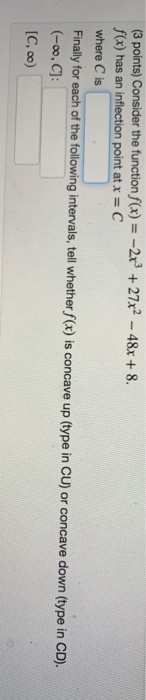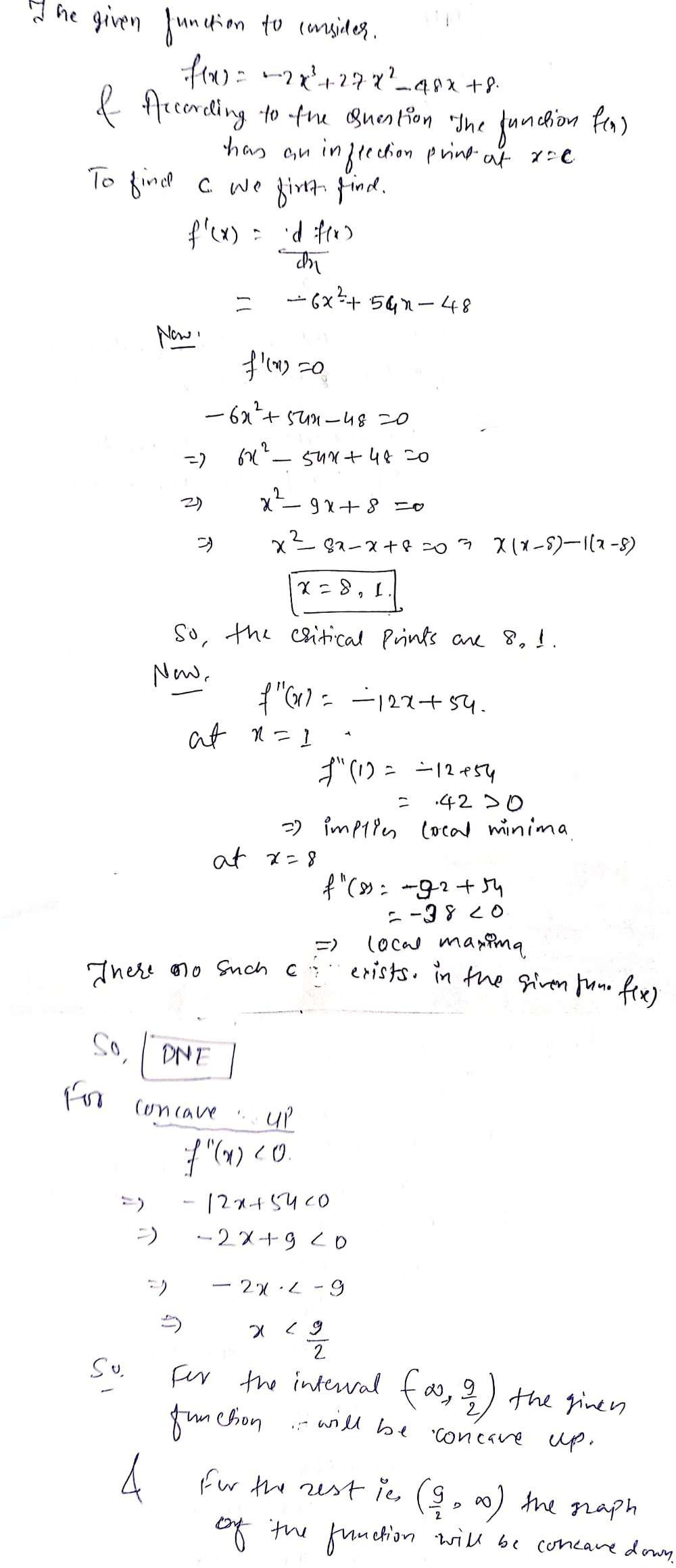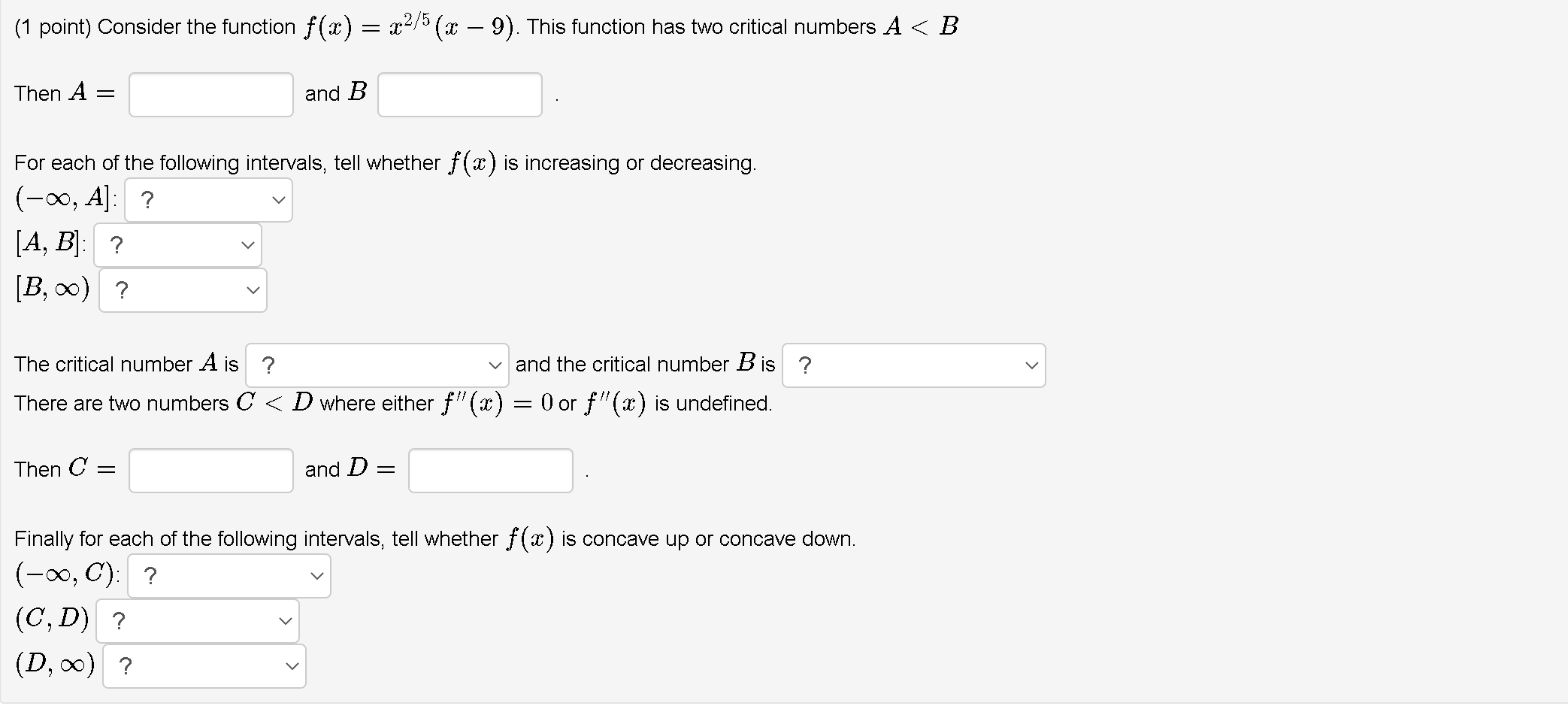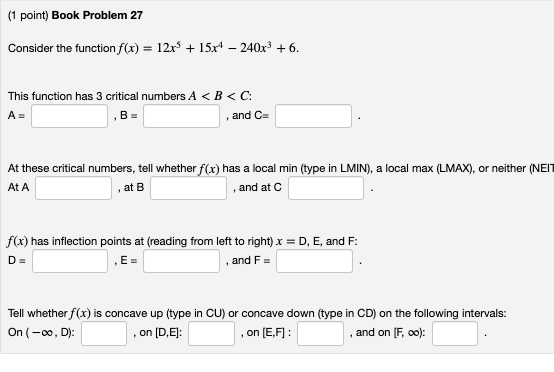# (3 points) Consider the function f(x) = -2x + 27x? - 48x + 8. f(x) has...(3 points) Consider the function f(x) = -2x + 27x? - 48x + 8. f(x) has an inflection point at x = C where is Finally for each of the following intervals, tell whether f(x) is concave up (type in CU) or concave down (type in CD). (-0,C]: [C,00)##### Add Answer to: (3 points) Consider the function f(x) = -2x + 27x? - 48x + 8. f(x) has...
Similar Homework Help Questions
• ### Consider the function f(x)= (2x+8)/(6x+3)

Consider the function f(x)= (2x+8)/(6x+3). For this function there are two important intervals: (-inf, A) (A, inf) and where the function is not defined at A . Find AFor each of the following intervals, tell whether is increasing (type in INC) or decreasing (type in DEC). Note that this function has no inflection points, but we can still consider its concavity. For each of the following intervals, tell whether is concave up (type in CU) or concave down (type in...

• ### Critical Points Calculus

Consider the function f(x) = 5 x + 5 x ^ { -1 }. For this function there are four important intervals: (-infty, A], [A,B),(B,C], and [C,infty) where A, and C are thecritical numbers and the function is not defined at B.Find A:and B:and C:For each of the following intervals, tell whether f(x) is increasing (type in INC) or decreasing (type in DEC).(-infty, A]:[A,B):(B,C]:[C,infty):Note that this function has no inflection points, but we can still consider its concavity. For each...

• ### Critical inflection step problem

Consider the function f(x)=8x+8x−1. For this function there are four important intervals: (−∞,A], [A,B),(B,C], and [C,∞) where A, and C are the criticalnumbers and the function is not defined at B.Find A ___________and B ____________and C ______________For each of the following intervals, tell whether f(x) is increasing (type in INC) or decreasing (type in DEC).(−∞,A]: _____________[A,B): ___________(B,C]: ________[C,∞) :________________Note that this function has no inflection points, but we can still consider its concavity. For each of the following intervals, tell...

• ### Consider the function f(x) = 4(x - 2)2/3. For this function there are two important intervals:...Consider the function f(x) = 4(x - 2)2/3. For this function there are two important intervals: (- 00, A) and (A, 0c) where A is a critical number. Ais For each of the following intervals, tell whether f(x) is increasing or decreasing. (-0, A): Select an answer v (A, 0): Select an answer v For each of the following intervals, tell whether f(x) is concave up or concave down. (- 00, A): Select an answer (A, 00): Select an answer

• ### inflections

part 1Consider the function . For this function there are four important intervals: , ,, and where , and arethe critical numbers and the function is not defined at .Find and and For each of the following intervals, tell whether is increasing (type in INC) or decreasing (type in DEC).::::part2Consider the function . has two inflection points at x = Cand x = D with where isand isFinally for each of the following intervals, tell whether is concave up (type...

• ### (1 point) Consider the function f(x) = x2/5(x – 9). This function has two critical numbers...(1 point) Consider the function f(x) = x2/5(x – 9). This function has two critical numbers A< B Then A = and B For each of the following intervals, tell whether f(x) is increasing or decreasing. (-0, A]: ? [A, B]: ? [B, 0) ? The critical number A is ? and the critical number B is ? There are two numbers C < D where either F"(x) = 0 or f'(x) is undefined. Then C= and D= Finally for...

• ### prob4Consider the function . has two inflectionpoints at x = C and x = D with where is_____________??___________ and is _____________??___________ Finally for each of the following intervals, tell whether is concave up (type in CU) orconcave down (type in CD). :_____________??___________ :_____________??___________ _____________??___________

• ### Concavity

Consider the function f(x) = 12 x^5 + 60 x^4 - 240 x^3 + 7.f(x) has inflection points at (reading from left to right) x = D, E, and Fwhere D is ___and E is ____and F is ____For each of the following intervals, tell whether f(x) is concave up (type in CU) or concave down (type in CD).(-infty, D]:[D,E]:[E,F]:[F,infty):

• ### Help please as soon as possibleConsider the function . For this function there are two importantintervals: and where thefunction is not definedat . Find For each of the following intervals, tell whether is increasing (type in INC) ordecreasing (type in DEC). : Note that this function has no inflection points, but we can stillconsider its concavity. For each of the following intervals, tellwhether is concave up (type in CU)orconcave down (type in CD). :

• ### Each blank is a part of one question so fill in all blanks please. (1 point)...Each blank is a part of one question so fill in all blanks please. (1 point) Book Problem 27 Consider the function f(x) = 12x5 + 15x4 - 240x3 + 6. This function has 3 critical numbers A <B<C: B = and C= A= At these critical numbers, tell whether f(x) has a local min (type in LMIN), a local max (LMAX), or neither (NEIT at B and at C At A f(x) has inflection points at (reading from left...

Free Homework App